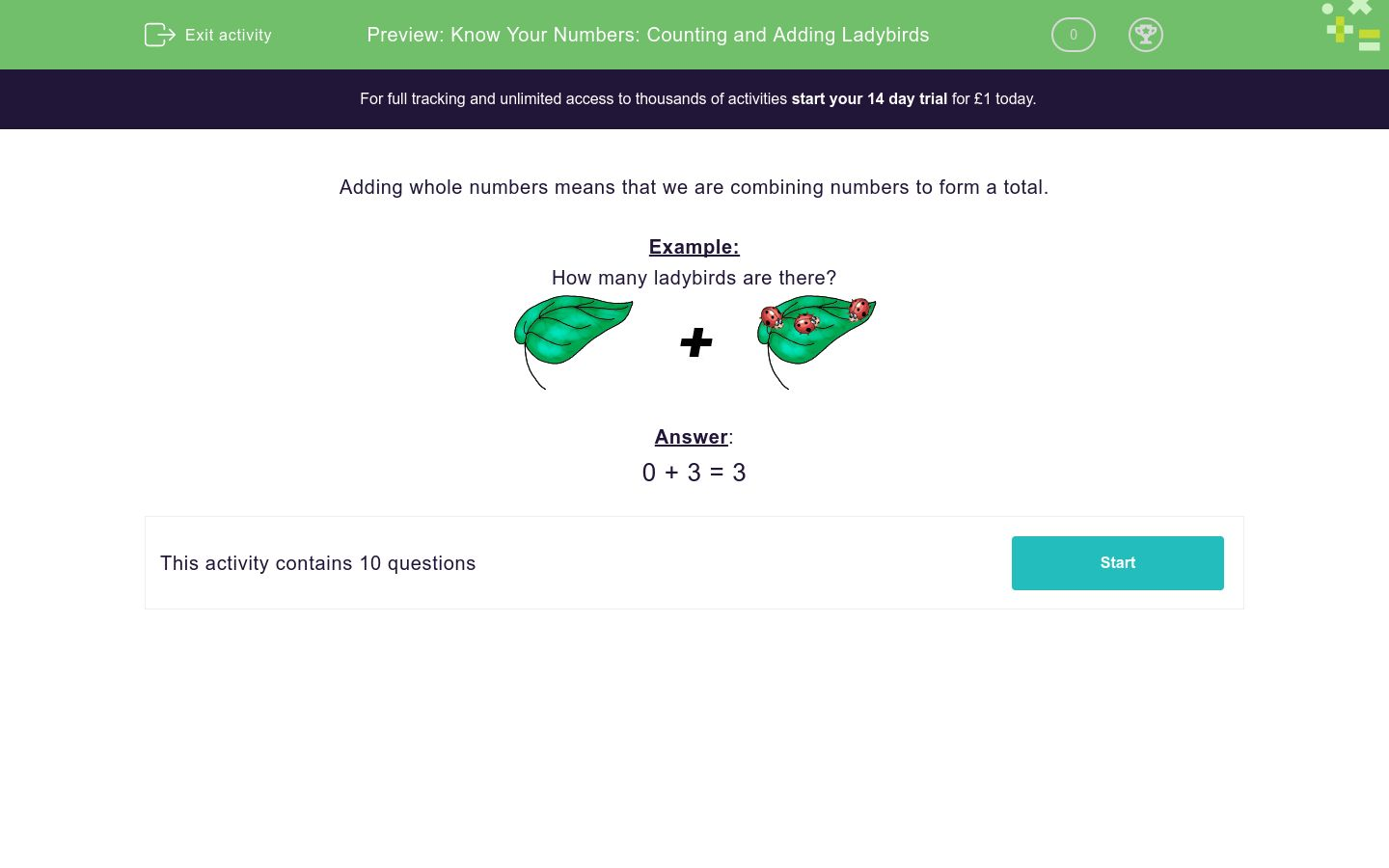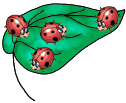### Affordable pricing from £10/month

In this worksheet, students count the number of ladybirds (in the number range 0-10) then add the numbers together.Key stage:  KS 1

Curriculum topic:   Number: Addition and Subtraction

Curriculum subtopic:   Make Mathematical Statements Using +, - and =

Difficulty level:### QUESTION 1 of 10

Adding whole numbers means that we are combining numbers to form a total.

Example:0 + 3 = 3• Question 13
EDDIE SAYS
3 + 0 = 3
• Question 24
• Question 34
EDDIE SAYS
2 + 2 = 4
• Question 41
• Question 58
EDDIE SAYS
4 + 4 = 8
• Question 67
EDDIE SAYS
4+3 = 7
• Question 75
EDDIE SAYS
1 + 4 = 5
• Question 86
EDDIE SAYS
2 + 1 + 3 = 6
• Question 99
EDDIE SAYS
4 + 3 + 2 = 9
• Question 106
EDDIE SAYS
1 + 4 + 1 = 6
---- OR ----

Sign up for a £1 trial so you can track and measure your child's progress on this activity.

### What is EdPlace?

We're your National Curriculum aligned online education content provider helping each child succeed in English, maths and science from year 1 to GCSE. With an EdPlace account you’ll be able to track and measure progress, helping each child achieve their best. We build confidence and attainment by personalising each child’s learning at a level that suits them.

Get started